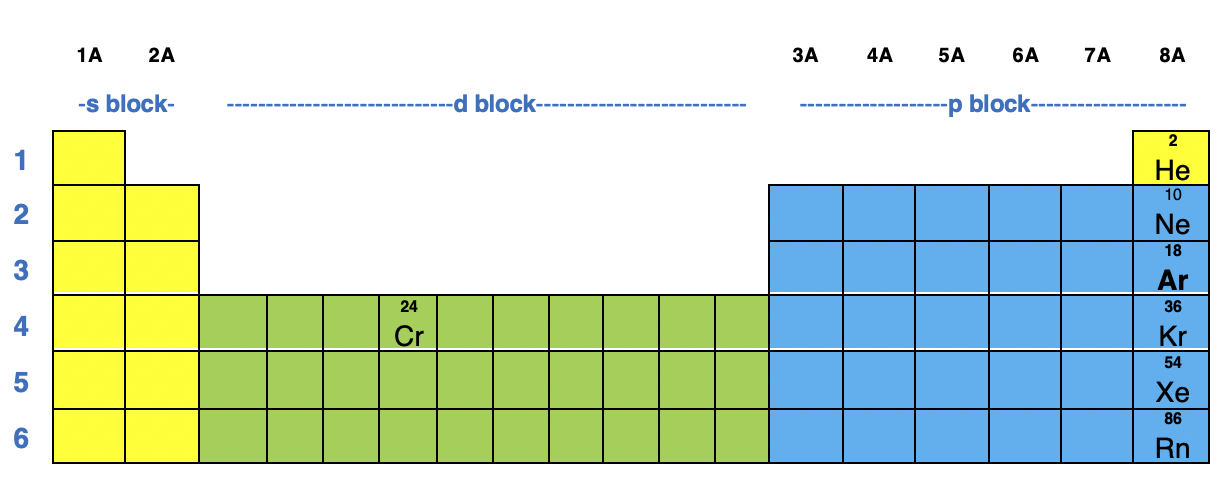# Problem: How many unpaired electrons would you expect on chromium in ammonium chromate?

###### FREE Expert Solution

Step 1: Recall that for a neutral element, Atomic number = # of protons = # of electrons

The atomic number of Cr is 24 which means Cr has 24 electrons.

The location of Cr in the periodic table is shown below:Based on the figure, Cr belongs in the d-block in Period 4 so its electron configuration will contain the 4s and 3d subshells

Recall that:

s–subshell can hold a maximum of 2 electrons

p–subshell can hold a maximum of 6 electrons

d–subshell can hold a maximum of 10 electrons

f–subshell can hold a maximum of 14 electrons

Starting from 1s, the electron configuration for Cr is:

Cr: 1s22s22p63s23p64s23d4

Step 2: In ammonium chromate, the charge of Cr is +6.

The +6 charge means 6 e were lost. Therefore, we need to subtract 3 e starting from the highest energy level (4s).

The electron configuration for Cr6+ is:

Cr6+: 1s22s22p63s23p6

81% (255 ratings)###### Problem Details

How many unpaired electrons would you expect on chromium in ammonium chromate?